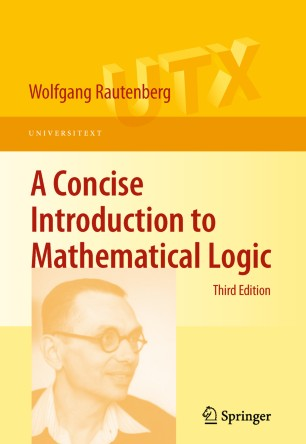# A Concise Introduction to Mathematical Logic

• Wolfgang RautenbergTextbook

Part of the Universitext book series (UTX)

1. Front Matter
Pages i-xxi
2. Wolfgang Rautenberg
Pages 1-40
3. Wolfgang Rautenberg
Pages 41-90
4. Wolfgang Rautenberg
Pages 91-134
5. Wolfgang Rautenberg
Pages 135-168
6. Wolfgang Rautenberg
Pages 169-214
7. Wolfgang Rautenberg
Pages 215-268
8. Wolfgang Rautenberg
Pages 269-298
9. Back Matter
Pages 299-319

### Introduction

Traditional logic as a part of philosophy is one of the oldest scientific disciplines and can be traced back to the Stoics and to Aristotle. Mathematical logic, however, is a relatively young discipline and arose from the endeavors of Peano, Frege, and others to create a logistic foundation for mathematics. It steadily developed during the twentieth century into a broad discipline with several sub-areas and numerous applications in mathematics, informatics, linguistics and philosophy.

This book treats the most important material in a concise and streamlined fashion. The third edition is a thorough and expanded revision of the former. Although the book is intended for use as a graduate text, the first three chapters can easily be read by undergraduates interested in mathematical logic. These initial chapters cover the material for an introductory course on mathematical logic, combined with applications of formalization techniques to set theory. Chapter 3 is partly of descriptive nature, providing a view towards algorithmic decision problems, automated theorem proving, non-standard models including non-standard analysis, and related topics.

The remaining chapters contain basic material on logic programming for logicians and computer scientists, model theory, recursion theory, Gödel’s Incompleteness Theorems, and applications of mathematical logic. Philosophical and foundational problems of mathematics are discussed throughout the text. Each section of the seven chapters ends with exercises some of which of importance for the text itself. There are hints to most of the exercises in a separate file Solution Hints to the Exercises which is not part of the book but is available from the author’s website.

### Keywords

Completeness theorems First-order Logic Incompleteness theorems Kurt Goedel Logical programming Mathematical logic Self-reference calculus logic mathematics recursion set theory theorem

#### Authors and affiliations

• Wolfgang Rautenberg
• 1
1. 1.Fachbereich Mathematik und Informatik, Institut für MathematikFreie Universität BerlinBerlinGermany

Industry Sectors